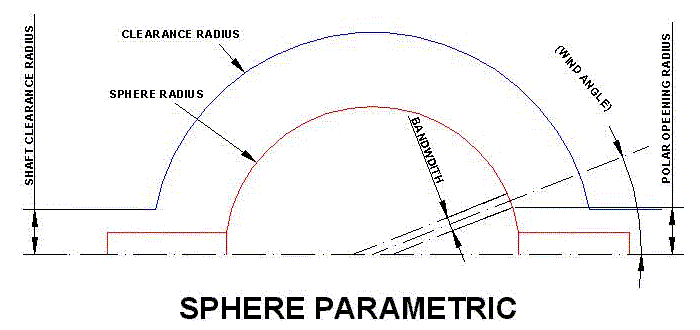# Sphere Parametric

## Overview

The sphere parametric automatically creates multiple layer winding programs to make a multi-angle layup on spherical components. The programs can give near uniform thickness distribution particularly if the filament bands are thin and a large number of layers are used. Because multiple wind angles are used the resulting composite is effectively in-plane quasi-isotropic and this gives maximum efficiency for a spherical pressure vessel. Ultra-high performance pressure vessels have been produced and tested using this method.The user defines the sphere geometry (radius and end opening radius) and material parameters such as the band width and thickness and the number of individual winding angles that are required. The program then creates all the necessary winding path information and transition paths that wind from the end point of one path to the start point of the next. The parametric program is invoked from the QuickCAD options of the Cadfil main menu.

The program generates a series of .PAY files for example if the parametric data was in the file SP.PAR the software would generate SP01.PAY, SP02.PAY, SP03.PAY....... A maximum of 99 payout files can be created. A special control (list) file SP.CTL would also be created. When the data is post-processed the .ctl file identifies all the individual .PAY files such that they can be automatically processed in sequence to create a single NC winding program.

A combined winding ( 'job-name'.CTL ) file is automatically created that includes all the .pay files. This can be post-processed to make the full winding program or used in the View Multiple payout files option to see the complete winding. Thickness files (.TH2) for each layer are also automatically created as well as a thickness list file 'jobname'_th2_lst.txt. This can be used as an input in the option to create finite element analysis output.### Band Width

\$SPH-BANDWIDTH is the true band width of the fibre bundle/tape.

### X- End opening

\$SPH-END-OP-RAD-LH is the radius of the end opening at the X- (left) end, where no winding takes place. Normally the mandrel would have a boss or fill tube.

### X+ End opening

\$SPH-END-OP-RAD-RH is the radius of the end opening at the X+ (right) end, where no winding takes place. This can be set to 0 if there is only an opening at one end.

### Number of layers/angles

\$SPH-NO-FW-LAYERSis the number of different angles which are required. For an even thickness distribution, this can be calculated by dividing a quarter of the spherical circumference by the bandwidth.

### Number of points/circuit

\$SPH-PTS-PER-CIRC. Each winding program has a number of data points on it and this is the number of points per circuit. A value of 20 works well in most applications. This is a compromise between accuracy and the amount of data created.

\$SPH-SURF-CLEARANCE is the clearance value for the machine. This should include the mandrel radius, and therefore the value should always be greater than the Sphere radius defined above.

### X- End shaft clearance radius

\$SPH-CLR-SHAFT-LH is the required machine clearance from the X- (left shaft)

### X+ End shaft clearance radius

\$SPH-CLR-SHAFT-RH is the required machine clearance from the X+ (right shaft)

### Band Thickness t0

\$SPH-PLY-T0 is the thickness of an individual fibre band used for thickness calculations.

### Winding angle list

\$SPH-WIND-ANG-LIST1 is a list of required winding angles if this is required. This line is normally left empty as the winding angles are automatically calculated from the number of layers/band sets. If used these values and wind angles (in degrees) commas or spaces. e.g. 10.0,15.0,20.0,25.0

### Winding angle list

\$SPH-WIND-ANG-LIST2 is a list of required winding angles if this is required. these will take the appearance of numbers separated by commas or spaces. This second line is available in case there is not enough space on the first line. The three lines are combined when the calculations occur.

### Winding angle list

\$SPH-WIND-ANG-LIST3 is a list of required winding angles if this is required. these will take the appearance of numbers separated by commas or spaces. This third line is available in case there is not enough space on the second line. The three lines are combined when the calculations occur.